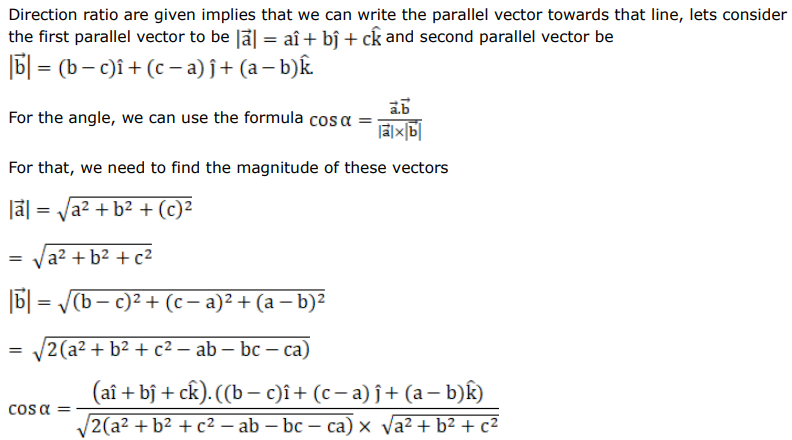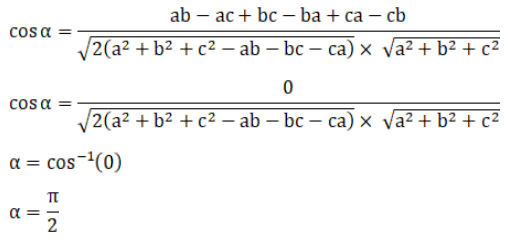# The direction ratios of two lines areQuestion:

The direction ratios of two lines are $a, b, c$ and $(b-c),(c-a),(a-b)$ respectively. The angle between these lines is

A. $\frac{\pi}{3}$

B. $\frac{\pi}{2}$

C. $\frac{\pi}{4}$

D. $\frac{3 \pi}{4}$

Solution: